# How to Do Beam Load Calculations

Page content

Just a look around will relate to us the simple, yet interesting fact that each and every object, living or non-living, is constantly applying a certain load over a certain base and also simultaneously is being subjected to an equal and opposite magnitude of force by the supported base.

A car parked over a place exerts a force or offers a load on the ground that may be equal to its weight; however the ground also presents an equal but opposite force to the car so that it stays in place intact. Since the car is held at one constant position, this implies that the two forces must be equal and acting in opposite directions.

Basically the following two forces normally act over any object that fundamentally constitute a load:

• Weight of the object, acting toward the ground
• Reaction of the ground or the base, acting upwards over the object

Before we move into the details of beam load calculations, it would be important to first know about types of loads that may act over a beam supported at its ends.

A load may be classified into the following important types:

• Point load, confined sharply over a single point,
• Equally or uniformly distributed load and,

Let’s understand them one by one.

Point Load: A load or weight subjected over a point area is called a point load. However, mathematically a point load doesn’t look feasible, simply because any load will need to have a certain area of impact and cannot possibly balance over a point, but if the impact area is too small compared to the length of the beam, may be taken as defined.

Equally Distributed Load: As the name implies a load that’s equally aligned across the whole beam is termed an equally distributed load.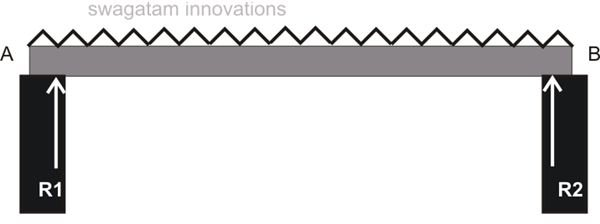Uniformly Varying Load: Loads distributed over a beam which produces a uniformly increasing load gradient across the entire beam end to end is called a uniformly varying load.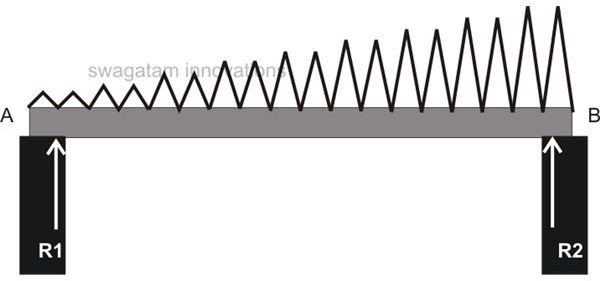A beam possibly may be subjected to one of the above loads or in combinations.

## Beam Reactions

The following simple illustration will walk us through formulas relating to beam load calculations or, more accurately, beam reactions: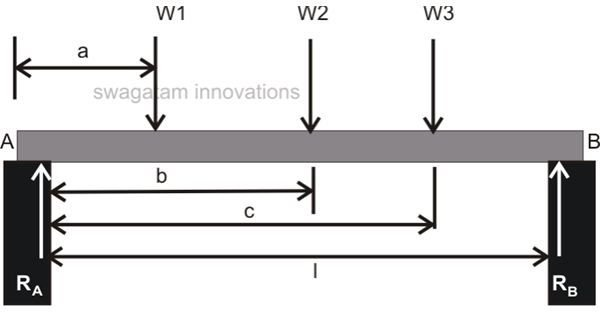Referring to the diagram alongside, let’s consider a beam being supported at its ends (left and right), denoted by the letters A and B respectively.

Let there be point loads acting on the beam over positions marked as W1, W2, and W3.

Also let,

RA = Reaction at the end A of the beam.

RB = Reaction at the end B of the beam.

Now, there are primarily a couple of forces (turning effect) that’s acting over the beam ends A and B viz. clockwise and anti-clockwise moment of force.

Since the moment of force over a supported beam is equal to the product of Force (weight here) and its distance from the support or the pivot, total clockwise moment acting at point A may be given as:

W1.a + W2.b + W3.c,

Also, the anticlockwise moment of force acting over point B must be:

RB.l

Now because the beam is in equilibrium, implies that the above two moments of force must be equal in magnitudes, therefore equating the two expressions gives:

W1.a + W2.b + W3.c = RB.l

RB = W1.a + W2.b + W3.c / l

The equilibrium with the beam also implies that:

RA + RB = W1.a + W2.b + W3.c

RA = ( W1.a + W2.b + W3.c) - RB

Now as per the conditions of equilibrium, the algebraic sum of all horizontal components in the above expression becomes immaterial and can be nullified (ƩH = 0.)

Therefore, the final equation becomes

RA = ( W1 + W2 + W3) - RB

The above formula may be used for finding out the reaction of a loaded beam about its end supports.

## Calculating Shear Force and Bending Moment

The two important parameters also involved with beam load calculations are Shear Force (SF) and Bending Moment (BM).

Let’s derive them with the help of the following simple illustration: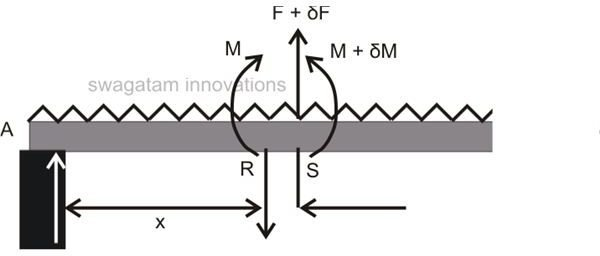Referring to the figure alongside, consider a beam loaded with uniformly distributed load of W per unit length. Also, consider a certain section of the beam RS, having a length δx at a distance x from the LHS (Left Hand Side) support of the beam.

The load acting over the section RS of the beam will be equal to W. δx (moment of Force).

Now assuming the Shear Force at point R to be = F,

Then at point S it will be F + δF.

Also, if the Bending Moment at R = M, then at S it becomes M + δM.

Since the beam is at equilibrium, the involved moment must also follow the laws of equilibrium, therefore equating two unbalanced expressions at S we get:

_F + W._δx = F + δF

Or δF/δx = W,

The above expressions indicate that the rate of change of Shear Force is equal to the load pressure or the intensity.

Similarly the moments at S may be equated as:

M – F.δx – Wδx2/2 = M + δM

Or δM = – F.δx, (ignoring the trivial quantity δx2)

We get, δM/δx = – F

The above relation shows that the rate of change of the Bending Moment is equal to the Shear Force of the section RS.

The data (Reaction formula, Shear Force and Bending Moment Relation) explained in this article may be used during beam load calculations for further determining the quality and the type of material to be used for loading the beam safely.

## Measuring Bending Moment in Cantilever Beams

A beam fixed at one end and freely hanging at the other is called a cantilever beam.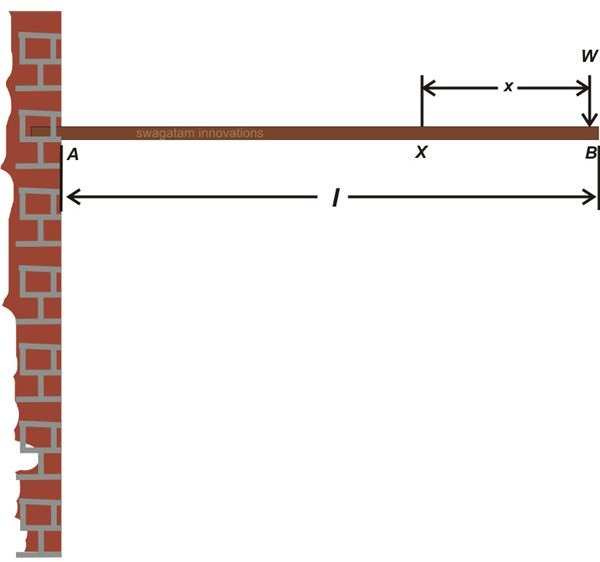Looking at the figure shown in this section, consider a cantilever beam having a length l and supporting a load W over its free end_._ Examining the section _X_ which is at a distance _x_ from the free end we find that the shear force equals the total unbalanced force (weight) acting vertically over the beam, i.e.:

Fx = –W (the minus sign is due to right side going downwards)

And the bending moment can be expressed as: